# Ignoring Blank or Zero Cells with Conditional formatting

This post will guide you how to make conditional formatting ignore blank cells or zero cell in Excel. How do I force blank cells or zero cells to be ignored in conditional formatting in Excel. How to ignore blank cells or blank cells when applying conditional formatting in Excel.

## Ignoring Blank with Conditional Formatting

When you create conditional formatting rules for a list of selected data, and you want to ignore all Blank cells, How to achieve it. Just do the following steps:

#1 select the source data that you want to apply the conditional Formatting.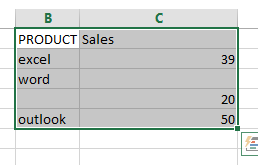#2 go to HOME tab, click Conditional Formatting command under Styles group. And select New Rule from the popup menu list. And the New Formatting Rule dialog will open.

#3 select Use a formula to determine which cells to format in the Select a Rule Type section.

#4 type the following formula into the Format values where this formula is true text box. Click Ok button.

=ISBLANK(B1)=TRUE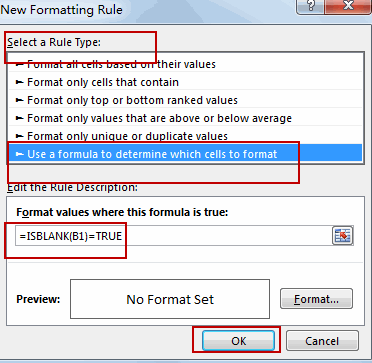Note: the B1 is the first cell of the selected range of cells.

## Ignoring zero with Conditional Formatting

If you want to ignore zero values with conditional Formatting, you can do the following steps:

#1 select the range of cells C2:C5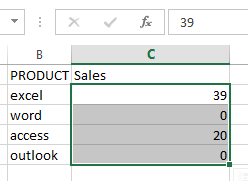#2 go to HOME tab, click Conditional Formatting command under Styles group, and select New Rule, and the New Formatting Rule dialog will open.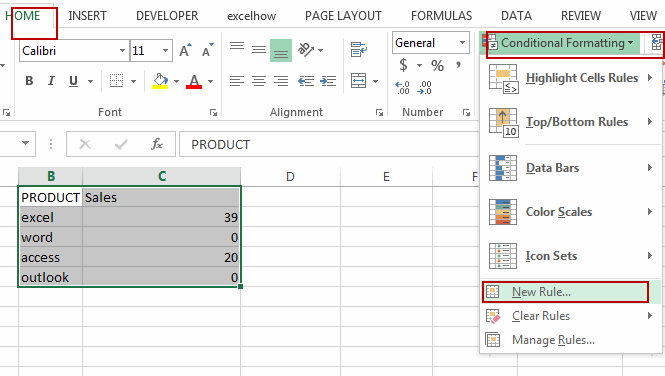#3 select Use a formula to determine which cells to format in the Select a Rule Type section.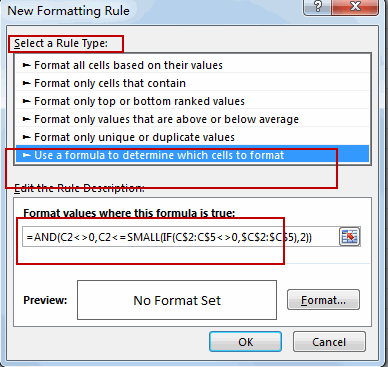#4 type the following formula into the Format values where this formula is true text box.

=AND(C2<>0,C2<=SMALL(IF(C\$2:C\$5<>0,\$C\$2:\$C\$5),2))

#5 click Format button, and switch to Fill tab in Format Cells dialog, select one color as the background color. Click Ok button.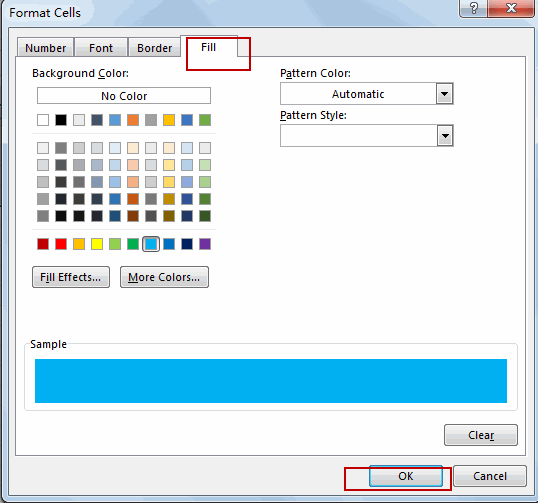#6 click Ok button. You will see that all zero values are ignored by conditional formatting.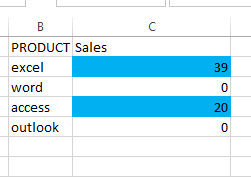### Related Functions

• Excel ISBLANK function
The Excel ISBLANK function returns TRUE if the value is blank or null.The syntax of the ISBLANK function is as below:= ISBLANK (value)…
• Excel IF function
The Excel IF function perform a logical test to return one value if the condition is TRUE and return another value if the condition is FALSE. The IF function is a build-in function in Microsoft Excel and it is categorized as a Logical Function.The syntax of the IF function is as below:= IF (condition, [true_value], [false_value])….
• Excel SMALL function
• The Excel SMALL function returns the smallest numeric value from the numbers that you provided. Or returns the smallest value in the array.The syntax of the SMALL function is as below:=SMALL(array,nth) …
• Excel AND function
• The Excel AND function returns TRUE if all of arguments are TRUE, and it returns FALSE if any of arguments are FALSE.The syntax of the AND function is as below:= AND (condition1,[condition2],…)…
Related Posts

Extract matching values From Two Lists

Suppose that you are working with two lists containing few values, and you want to extract the matching values from those two lists into another separate list. You might prefer to manually extract the matching values from the two lists, ...

Extract all Partial Matches

Just assume that you have a range of data that contains a text string and you want to extract the few partial matches into another separate range of cells; then you might think that it's not a big deal; because ...

Extract Multiple Match Values into Separate Columns

If you have a few values/items in the excel sheet and you are thinking that with the aid of the “VlOOKUP” function you can look for a specific value, extract it and then put the matching item into the separate ...

How to Average Only Positive or Negative Numbers of a Range

Suppose both positive numbers and negative numbers exist in a table. If we want to know the average of only positive numbers in this table, we can create a formula to get average of all positive numbers with all negative ...

How to Sum the Smallest N Values in Excel

Sometimes we may want to sum the first smallest N numbers in a range in Excel. In this article, we will show you the method of “SUM the Smallest N Numbers” by a simple formula which consist of SUMPRODUCT and ...

How to Create Dynamical Drop-Down List and Sort by Alphabetical Order in Excel

In our daily work we may need to create a dynamical dropdown list and sort all values by alphabetical order. To create a dropdown list like this, we need to apply some built-in features like ‘Define Name’ and ‘Data Validation’ ...

How to Find the Smallest Value and Smallest Positive Value in Excel

Sometimes we want to find out the smallest positive value among a set of values. We should ignore the negative value and zero value when getting the smallest positive value. So we design different cases in this article to demonstrate ...

How to Get the First, 2nd or Nth Match Using Vlookup/Index/Match

This post will guide you how get the nth matching values with VLOOKUP function in Excel. How do I find the nth match value with Index/Match formula in Excel. How to find the first, second, third or nth matching value ...

Find the Earliest and Latest Date in a Range of Dates in Excel

This post will guide you how to find the earliest date in a range of dates in Excel. How do I get the earliest and latest date in a range with a formula in Excel. How to find the highest ...

Find Closest Value or Nearest Value in a Range in Excel

This post will guide you how to find the closest value or nearest value in a range of cells or in a column in Excel. How do I find the closest match in a range to a given lookup value ...

Sidebar